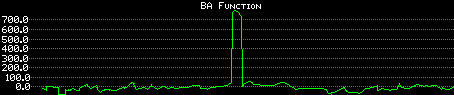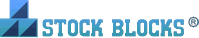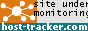# How the Box Analyzer reveals volume errors

## Q:

On one particular stock the BA FUNCTION window will show a flat line on the bottom of the window with a single spike at some arbitrary location. Why does this graph look like this and is there some setting I should use to make this more relevant? It doesn't seem to effect the placing of the BA BUY signals but I would like to know if there is anything wrong.## A:

This anomaly is observed for days whose boxes are extraordinarily "thinner" than their preceding boxes, and most likely shows that one of your days has a volume value that is incorrect due to a scaling error.

Check your tabulated data at the point where the "spike" begins. The volume value may be missing a couple of digits, causing it to be much smaller than its neighboring values, as in the following example (DATE, HIGH, LOW, VOLUME) ...

```9-6-1999, 18.25, 17.75, 54750
9-7-1999, 18.5, 17.50, 60736
9-8-1999, 18.5, 18.25, 282
9-9-1999, 19.75, 17.5, 107620```
As shown above, all data is fairly consistent with the exception of the third line, whose volume (value 282) appears to be off by a factor of 100. Here's why the BA function "explodes" in these situations: The BA data points are calculated by the following formula:
BA = (normalized box ratio) multiplied by (% midpoint move)

where:  "normalized box ratio" equals (normalized height)/(normalized width)

and...
"normalized height" reflects the high:low price gap
"normalized width" reflects the volume
So the smaller the normalized width is (that is, smaller volumes) the larger the normalized box ratio is (the thinner the box, the larger the box ratio). This results in amplifying the BA's magnitude for that day, which is exactly what you're observing. Whether the magnitude is strongly positive (as observed in the above figure) or negative depends on the sign (+/-) of the "% midpoint move".

So check your data. If you find that the volume is off by a factor of 100, then add two 0's to its end, then see how the BA changes.  It should then look fine.

You may wonder why zero-volume days don't produce disastrous effects with the BA (dividing by zero creates a box ratio equal to infinity!). Simply, the Box Analyzer ignores zero volume days in its calculations.Stock Blocks® Site Search All AnyP.O. Box 416, North Stonington CT 06359 USA